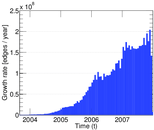# Delicious tag–item

This network contains tag–URL relations from http://delicious.com/. Left nodes represent tags, right nodes represent URLs and an edge shows that a URL was tagged with a tag.

 Code `Dti` Internal name `delicious-ti` Name Delicious tag–item Data source http://dai-labor.de/IRML/datasets AvailabilityDataset is available for download Consistency checkDataset passed all tests Category Feature network Node meaning Tag, URL Edge meaning Tag assignment Network formatBipartite, undirected Edge typeUnweighted, multiple edges Temporal dataEdges are annotated with timestamps Snapshot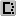Is a snapshot and likely to not contain all data

## Statistics

 Size n = 38,289,740 Left size n1 = 4,511,972 Right size n2 = 33,777,768 Volume m = 301,183,605 Unique edge count m̿ = 137,240,382 Wedge count s = 6,122,567,080,440 Claw count z = 899,959,427,749,358,592 Cross count x = 1.464 61 × 1023 Maximum degree dmax = 4,358,622 Maximum left degree d1max = 4,358,622 Maximum right degree d2max = 100,623 Average degree d = 15.731 8 Average left degree d1 = 66.752 1 Average right degree d2 = 8.916 62 Fill p = 9.005 02 × 10−7 Average edge multiplicity m̃ = 2.194 57 Size of LCC N = 37,307,789 Diameter δ = 26 50-Percentile effective diameter δ0.5 = 3.846 86 90-Percentile effective diameter δ0.9 = 5.348 70 Mean distance δm = 4.549 22 Gini coefficient G = 0.888 999 Balanced inequality ratio P = 0.128 759 Left balanced inequality ratio P1 = 0.045 446 5 Right balanced inequality ratio P2 = 0.191 702 Relative edge distribution entropy Her = 0.704 929 Power law exponent γ = 4.557 04 Degree assortativity ρ = −0.048 865 6 Degree assortativity p-value pρ = 0.000 00 Spectral norm α = 29,314.6

## Plots

### Degree distribution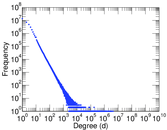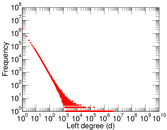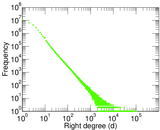### Cumulative degree distribution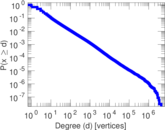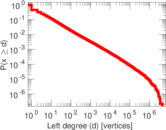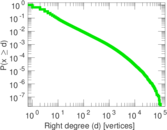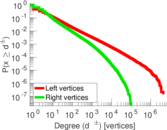### Lorenz curve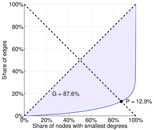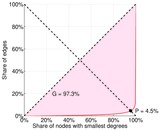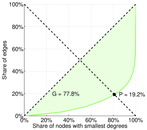### Spectral distribution of the adjacency matrix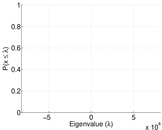### Spectral distribution of the normalized adjacency matrix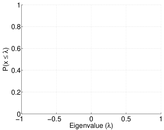### Spectral distribution of the Laplacian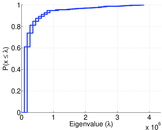### Spectral graph drawing based on the adjacency matrix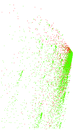### Spectral graph drawing based on the normalized adjacency matrix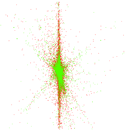### Degree assortativity### Hop distribution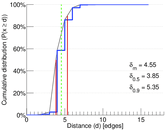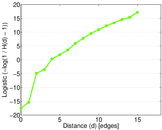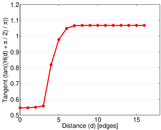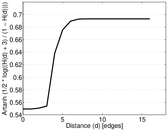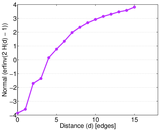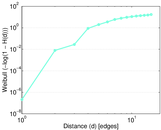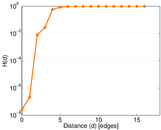### Edge weight/multiplicity distribution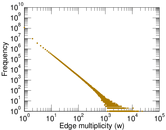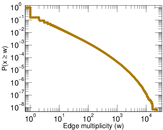### Temporal distribution